Draw consistently with various backends

# Pero

The main motivation behind the pero library is to provide unified API for multiple drawing backends like PyQt5, PyQt6, PySide2, PySide6, wxPython, PyCairo, PyMuPDF, Pythonista (and possibly more), which is easy to understand and use. Beside the common drawing capabilities, numerous pre-build glyphs are available, as well as an easy-to-use path, matrix transformations etc. Depending on available backend libraries, drawings can be viewed directly or exported into various image formats.

Ever since I discovered the wonderful d3js JavaScript library, I wanted to have the same amazing concept of dynamic properties within Python drawings. In fact, this has been the trigger to start working on the pero library. Finally, it is now available.

Consider also checking a small derived library providing some basic plotting functionalities, like profiles, bars, pie charts and Venn diagrams, called perrot.

import pero

img = pero.Image(width=200, height=200)

img.line_cap = pero.ROUND
img.line_join = pero.ROUND

# fill
img.fill("w")

# body
img.line_width = 2
img.line_color = pero.colors.Orange.darker(.1)
img.fill_color = pero.colors.Orange
img.draw_circle(100, 100, 75)

img.line_color = None
img.fill_color = pero.colors.White.darker(.1)
img.draw_ellipse(100, 185, 70, 10)

# eyes
img.fill_color = pero.colors.Black
img.draw_circle(70, 85, 15)
img.draw_circle(130, 85, 15)

# eyebrows
img.line_color = pero.colors.Black
img.fill_color = None
img.line_width = 3

# mouth
img.line_width = 5

# highlight
img.line_color = pero.colors.Orange.lighter(.3)

# hat
path = pero.Path(pero.WINDING)
path.ellipse(100, 27, 40, 10)
path.ellipse(100, 17, 30, 10)
path.rect(85, 17, 30, 10)

path.transform(mat)

img.line_color = None
img.fill_color = pero.colors.Black
img.draw_path(path)

# show image
img.show()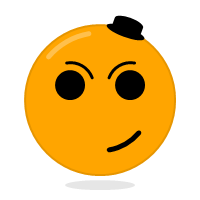## Installation

The pero library is fully implemented in Python. No additional compiler is necessary. After downloading the source code just run the following command from the pero folder:

$python setup.py install or simply use pip $ pip install pero

## Disclaimer

This program is distributed in the hope that it will be useful, but WITHOUT ANY WARRANTY; without even the implied warranty of MERCHANTABILITY or FITNESS FOR A PARTICULAR PURPOSE.

Please note that the pero library is still in an alpha state. Any changes in its API may occur.

## Usage

### Using default backend

If you just want to draw an image using whatever the default backend is (for requested format), or show the image directly (requires PyQt5, PyQt6, PySide2, PySide6, wxPython or Pythonista iOS App), just create an image and use it as any other pero canvas:

import pero

# init size
width = 200
height = 200

# init image
img = pero.Image(width=width, height=height)

# draw graphics
img.line_color = "b"
img.fill_color = "w"
img.fill()
img.draw_circle(100, 100, 75)

# save to file
img.export('image.png')

# show in viewer
img.show()


### Using PyQt5, PyQt6, PySide2 or PySide6

Inside a QWidget you can create a QPainter and encapsulate it into the pero canvas:

import pero
from PyQt5.QtGui import QPainter

# init size
width = 200
height = 200

# init painter
qp = QPainter()
qp.begin(self)
qp.setRenderHint(QPainter.RenderHint.Antialiasing)

# init canvas
canvas = pero.qt.QtCanvas(qp, width=width, height=height)

# draw graphics
canvas.line_color = "b"
canvas.fill_color = "w"
canvas.fill()
canvas.draw_circle(100, 100, 75)

# end drawing
qp.end()


### Using wxPython

Inside a wxApp you can use just about any wxDC you want and encapsulate it into the pero canvas:

import pero
import wx

# init size
width = 200
height = 200

# create DC
bitmap = wx.Bitmap(width, height)
dc = wx.MemoryDC()
dc.SelectObject(bitmap)

# use GCDC
if 'wxMac' not in wx.PlatformInfo:
dc = wx.GCDC(dc)

# init canvas
canvas = pero.wx.WXCanvas(dc, width=width, height=height)

# draw graphics
canvas.line_color = "b"
canvas.fill_color = "w"
canvas.fill()
canvas.draw_circle(100, 100, 75)


### Using PyCairo

Depending on the final image format, choose appropriate cairo surface, get the drawing context and encapsulate it into the pero canvas:

import pero
import cairo

# init size
width = 200
height = 200

# create cairo drawing context
surface = cairo.PSSurface('image.eps', width, height)
dc = cairo.Context(surface)

# init canvas
canvas = pero.cairo.CairoCanvas(dc, width=width, height=height)

# draw graphics
canvas.line_color = "b"
canvas.fill_color = "w"
canvas.fill()
canvas.draw_circle(100, 100, 75)

# save to file
dc.show_page()


### Using PyMuPDF

Create a document, add new page and encapsulate it into the pero canvas:

import pero
import fitz

# init size
width = 200
height = 200

# init document
doc = fitz.open()
page = doc.newPage(width=width, height=height)

# init canvas
canvas = pero.mupdf.MuPDFCanvas(page)

# draw graphics
canvas.line_color = "b"
canvas.fill_color = "w"
canvas.fill()
canvas.draw_circle(100, 100, 75)

# save to file
doc.save('image.pdf')
doc.close()


### Using SVG

The pero library implements its own way to draw and save SVG files. Just create a pero canvas:

import pero

# init size
width = 200
height = 200

# init canvas
canvas = pero.svg.SVGCanvas(width=width, height=height)

# draw graphics
canvas.line_color = "b"
canvas.fill_color = "w"
canvas.fill()
canvas.draw_circle(100, 100, 75)

# save to file
with open('test.svg', 'w', encoding='utf-8') as f:
f.write(canvas.get_xml())


### Using Pythonista

Initialize a new ui.ImageContext and create a pero canvas:

import pero
import ui

# init size
width = 200
height = 200

# open context
with ui.ImageContext(width, height) as ctx:

# init canvas
canvas = pero.pythonista.UICanvas(width=width, height=height)

# draw graphics
canvas.line_color = "b"
canvas.fill_color = "w"
canvas.fill()
canvas.draw_circle(100, 100, 75)

# show image
img = ctx.get_image()
img.show()


### Using glyphs and dynamic properties

Similar to d3js JavaScript library, most of the properties of pre-build pero.Glyphs objects can be specified as a function, to which given data source is automatically provided. Together with pero.scales (and maybe the pero.Axis) this can be used to make simple plots easily.

import pero
import numpy

# init size
width = 400
height = 300

# init data
x_data = numpy.linspace(-numpy.pi, numpy.pi, 50)
y_data = numpy.sin(x_data)

# init scales
x_scale = pero.LinScale(
in_range = (min(x_data), max(x_data)),

y_scale = pero.LinScale(
in_range = (-1, 1),

in_range = (-1, 1),
out_range = pero.colors.Spectral)

# init marker
marker = pero.Circle(
size = 8,
x = lambda d: x_scale.scale(d),
y = lambda d: y_scale.scale(d),
line_color = lambda d: color_scale.scale(d).darker(.2),
fill_color = lambda d: color_scale.scale(d))

# init image
image = pero.Image(width=width, height=height)

# fill
image.fill("w")

# draw points
marker.draw_many(image, zip(x_data, y_data))

# show image
image.show()


## Examples

In the examples folder you will find sample codes to generate and understand all the following images. Check the image name and find corresponding python draw file.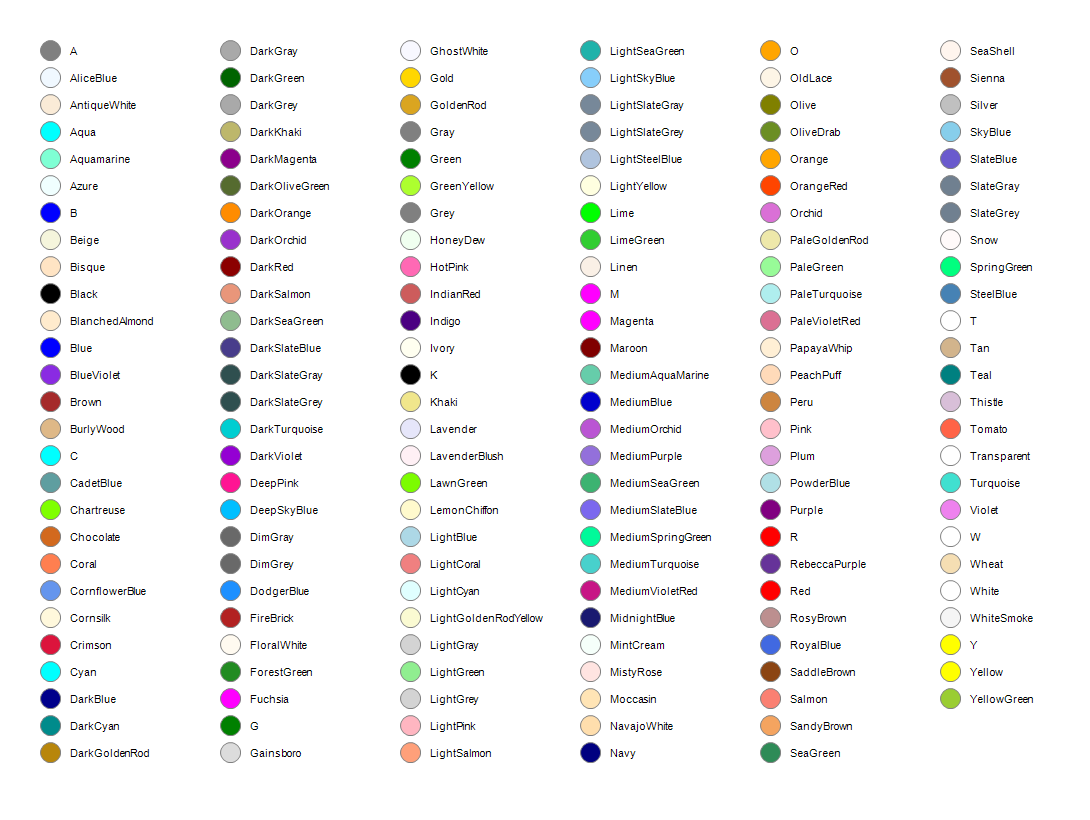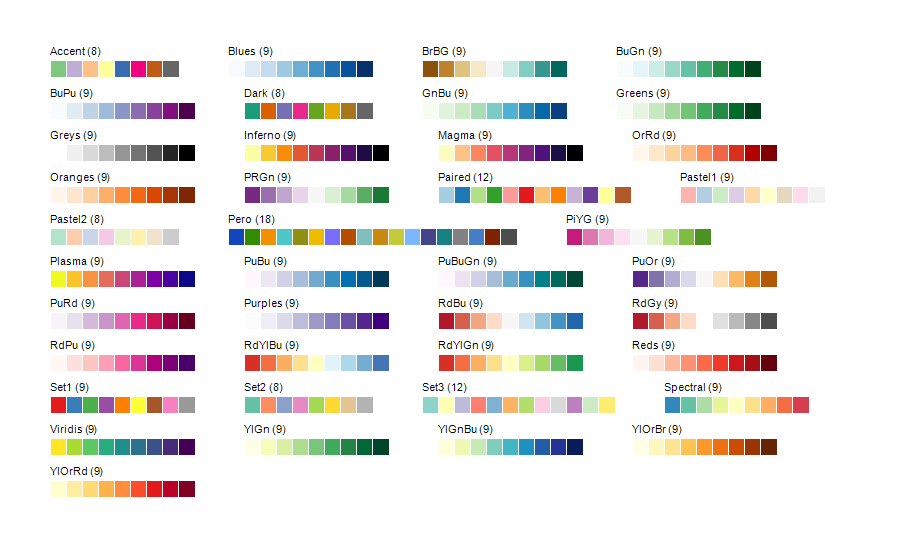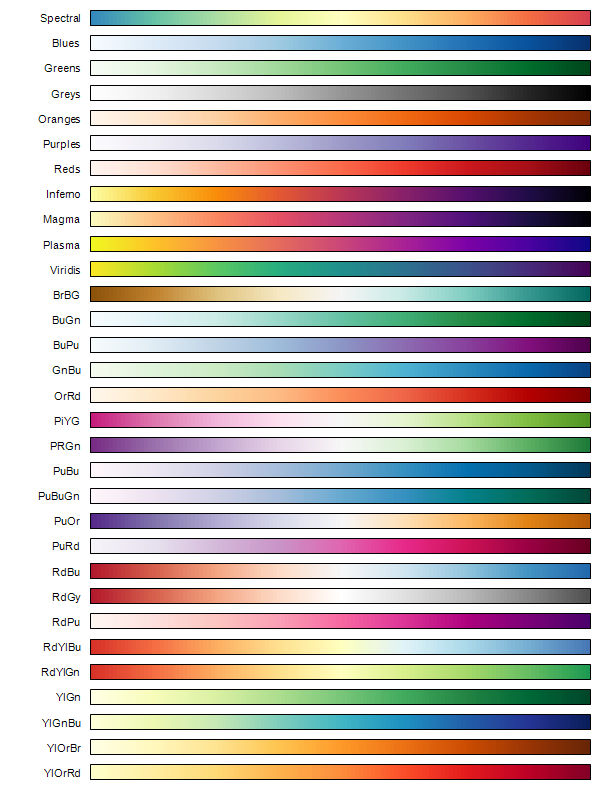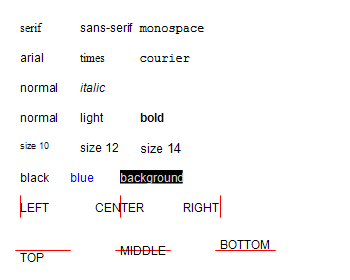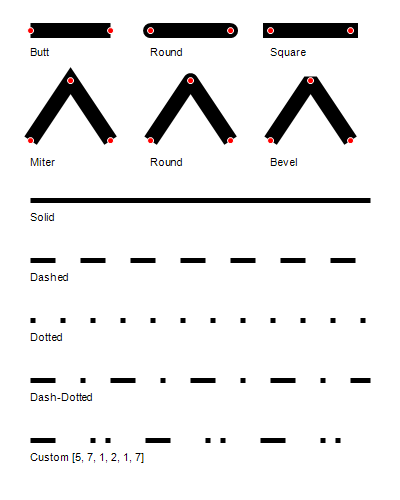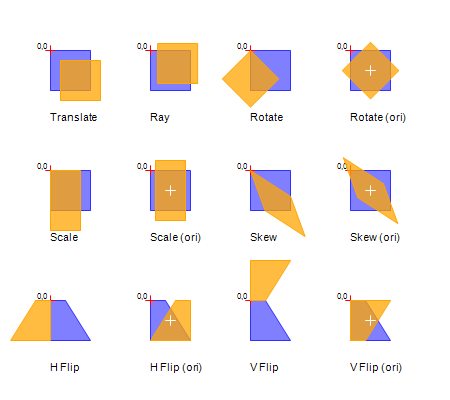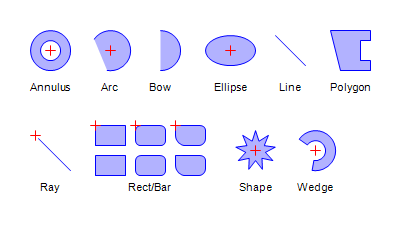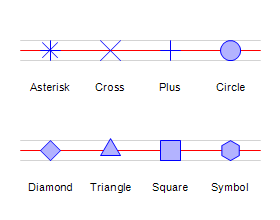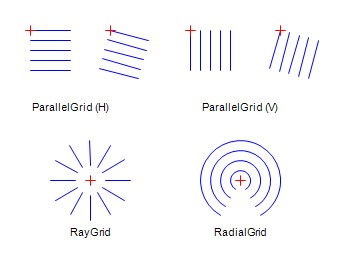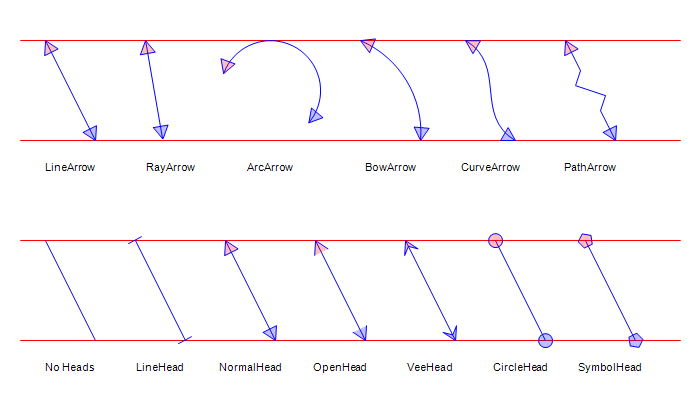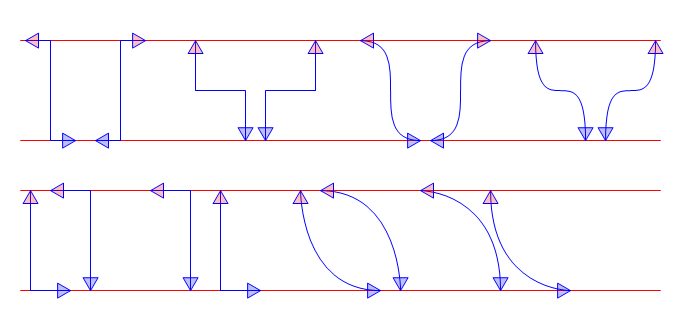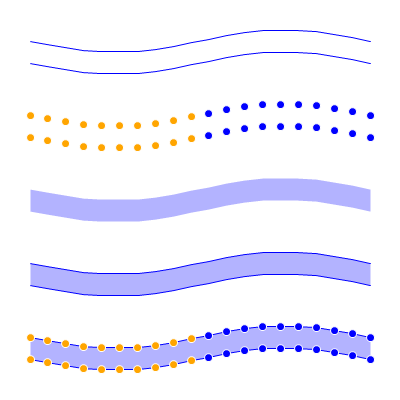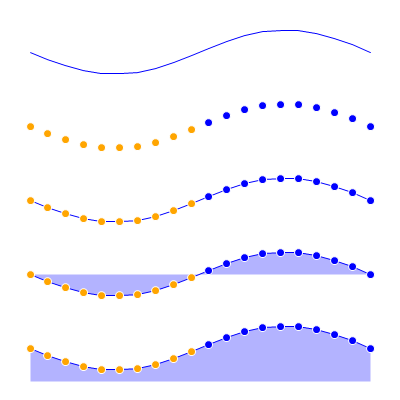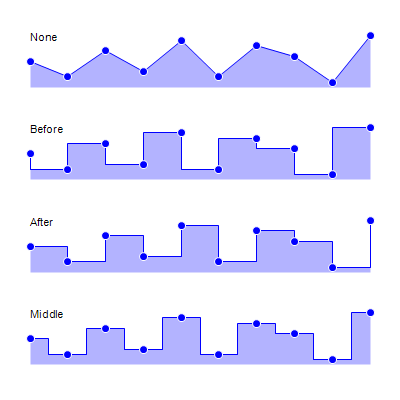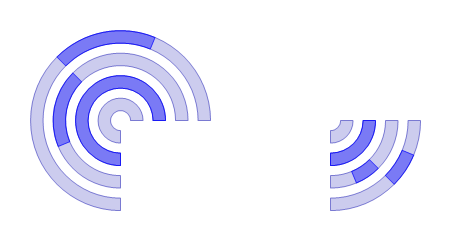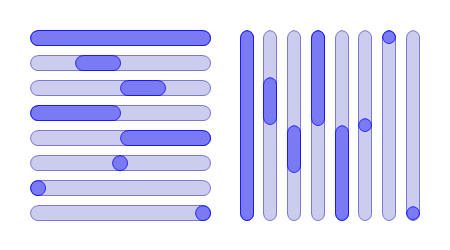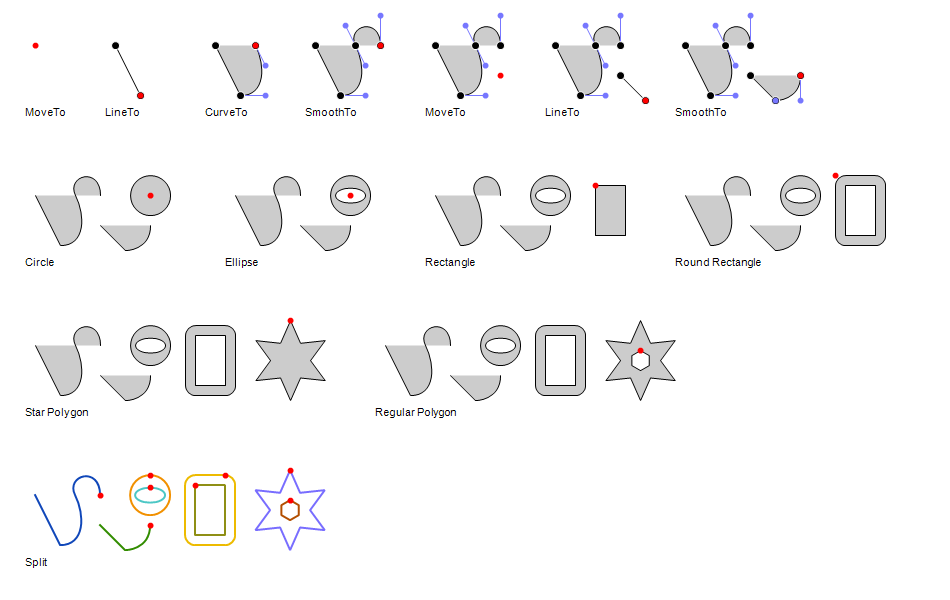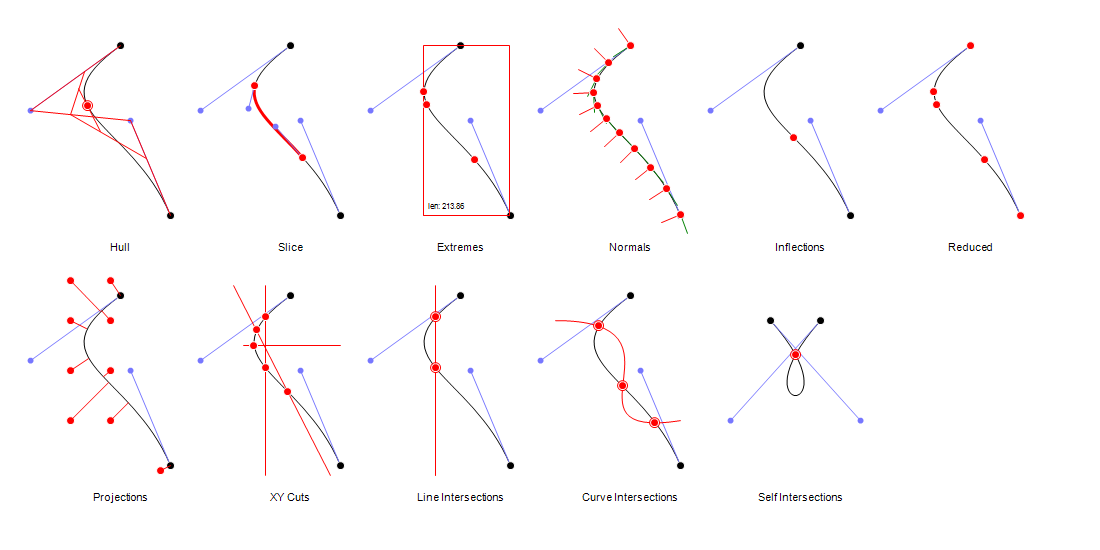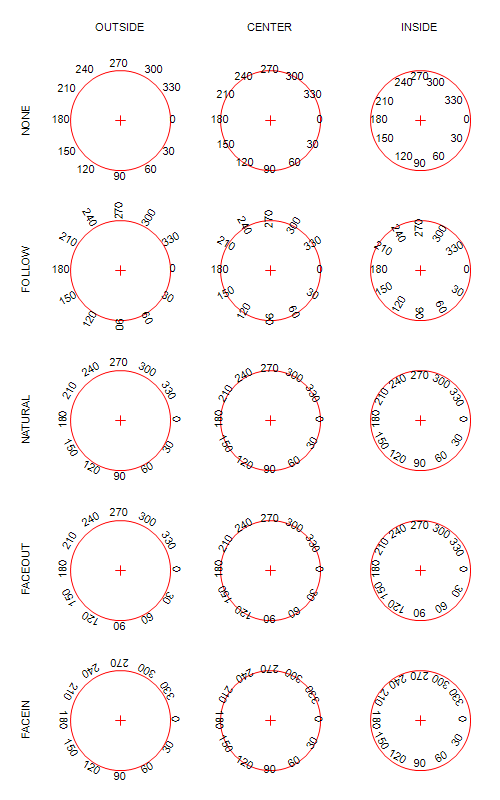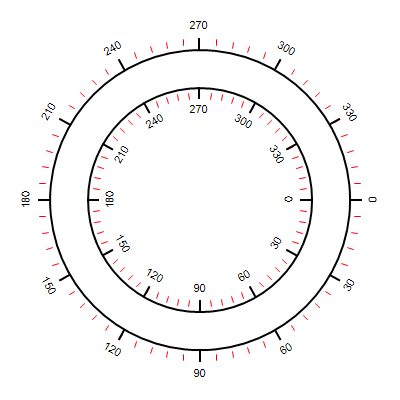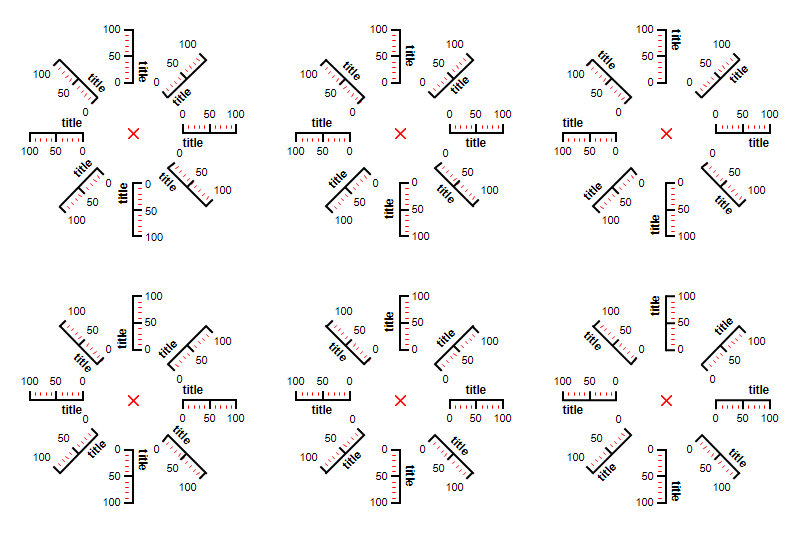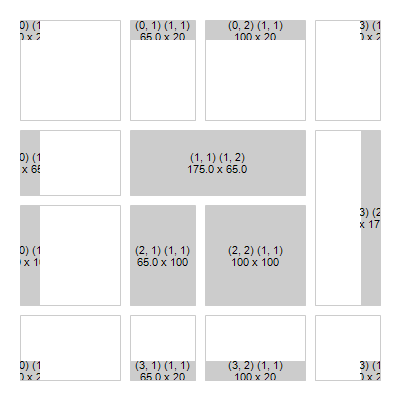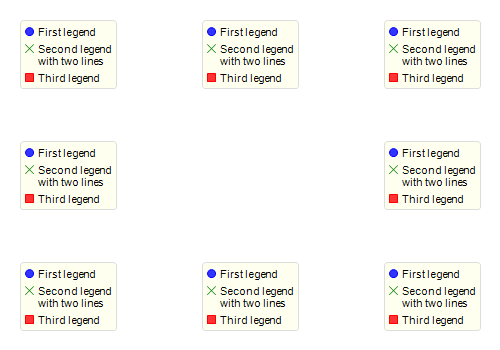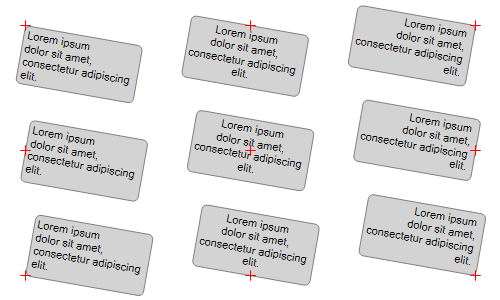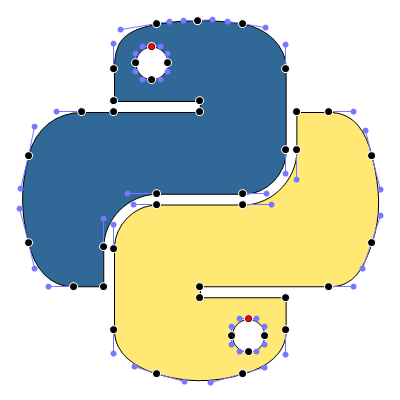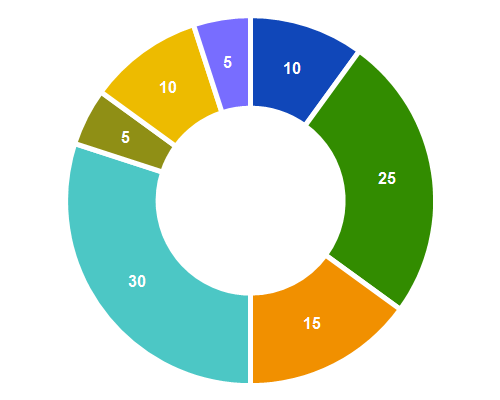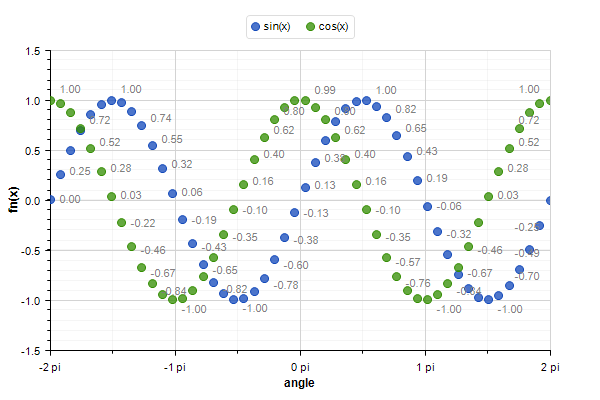## Project details

This version0.18.00.17.00.16.00.15.20.14.00.13.00.12.00.11.00.10.00.9.00.8.00.7.00.6.00.5.00.4.00.3.00.2.10.2.0

Uploaded source
Uploaded py3